Refer to our Texas Go Math Grade 6 Answer Key Pdf to score good marks in the exams. Test yourself by practicing the problems from Texas Go Math Grade 6 Lesson 16.1 Answer Key Area of Quadrilaterals.

Area of a Parallelogram

Recall that a rectangle is a special type of parallelogram.
A. Draw a large parallelogram on grid paper. Cut out your parallelogram.
B. Cut your parallelogram on the dashed line as shown. Then move the triangular piece to the other side of the parallelogram.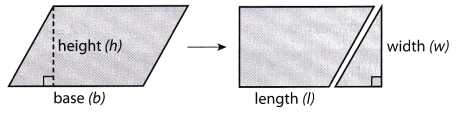C. What figure have you formed? ____________
Does this figure have the same area as the parallelogram? ____________
base of parallelogram = ____________ of rectangle
height of parallelogram = __________ of rectangle
area of parallelogram = __________ of rectangle
What is the formula for the area of this figure? A = ___________ or _________
D. What is the formula for the area of a parallelogram? A = ___________

Area of a Parallelogram
The area A of a parallelogram is the product of its base b and its height h.A = bh

Reflect

Question 1.
Find the area of the parallelogram.A = ____________
Data:
base = 14
height = 7
Write equation of area of a parallelogram:
Area = base × height
Substitute values:
Area = 14 × 7
Evaluate:
Area = 98
Area of the given parallelogram is 98 square centimeters.

Example 1

A section of a deck is in the shape of a trapezoid. What is the area of this section of the deck?
b1 = 17; b2 = 39; h = 16
Use the formula for area of a trapezoid.Question 2.
Another section of the deck is also shaped like a trapezoid. For this section, the length of one base is 27 feet, and the length of the other base is 34 feet. The height is 12 feet. What is the area of this section of the deck? A = _________ft2
Solution to this example is given below
b1 = 27; b2 = 34; h = 12
Use the formuLa for area of a trapezoid
A = $$\frac{1}{2}$$h(b1 + b2)
= $$\frac{1}{2}$$ ∙ 12(274 – 34) (Substitute)
= $$\frac{1}{2}$$ ∙ 12(61) (Add inside the parentheses)
= 6 ∙ 61 (Multiply $$\frac{1}{2}$$ and 12.)
= 366 square feet (Multiply)
= 366 ft2

Example 2

Cedric is constructing a kite in the shape of a rhombus. The spars of the kite measure 15 inches and 24 inches. How much fabric will Cedric need for the kite?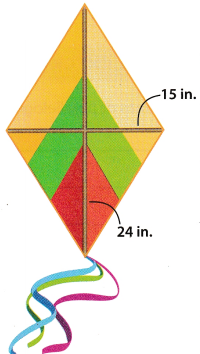To determine the amount of fabric needed, find the area of the kite.
d1 = 15; d2 = 24
Use the formula for area of a rhombus.
A = $$\frac{1}{2}$$d1d2
= $$\frac{1}{2}$$ (15) (24) Substitute.
= 80 square inches Multiply.

Find the area of each rhombus.

Question 3.
d1 = 35 m; d2 = 12 m
A = _________ m2
Find the area of a rhombus.
d1 = 35 m, and d2 = 12 m
Use the formula for area of a rhombuse.
A = $$\frac{1}{2}$$ d1d2
= $$\frac{1}{2}$$ (35) (12) (Substitute.)
= 210 square meters. (Multiply.)
The area is 210 square meters.
= 210 m2

Question 4.
d1 = 9.5 in.; d2 = 14 in.
A = ______ in2
Data:
d1 = 9.5
d2 = 14
Write equation of area of a rhombus:
Area = $$\frac{1}{2}$$ × d1 × d2
Substitute values:
Area = $$\frac{1}{2}$$ × 9.5 × 14
Evaluate:
Area = 66.5
Area of the given rhombus is 66.5 square inches.

Question 5.
d1 = 10 m; d2 = 18 m
A = ______ m2
Find the area of a rhombus.
d1 = 10 m, and d2 = 18 m
Use the formula for area of a rhombus.
A = $$\frac{1}{2}$$ d1d2
= $$\frac{1}{2}$$ (10) (18) Substitute.
= 90 square meters. Multiply.
The area is 90 square meters.
= 90 m2

Question 6.
d1 = 8$$\frac{1}{4}$$ ft; d2 = 40 ft
A = ________ ft2
$$8 \frac{1}{4}=\frac{8 \times 4+1}{4}$$ = 334 = 8.25 Convert to decimal number
Find the area of a rhombus.
d1 = 8.25 ft, and d2 = 40 ft
Use the formula for area of a rhombus.
A = $$\frac{1}{2}$$ d1d2
= $$\frac{1}{2}$$ (8.25)(40) Substitute.
= 165 square feet. Multiply
The area is 165 square feet
= 165 ft2

Question 1.
Find the area of the parallelogram. (Explore Activity)A = bh
= (________) (________)
= _______ in2.
Data:
b = 13
h = 9
Write equation of area of a parallelogram:
Area = b × h
Substitute values:
Area = 13 × 9
Evaluate:
Area = 117
Area of the given parallelogram is 117 square inches.

Question 2.
Find the area of the trapezoid. (Example 1)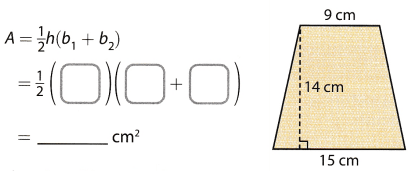Solution to this example is given below
b1 = 9; b2 = 15; h = 14
Use the formula for area of a trapezoid
A = $$\frac{1}{2}$$h(b1 + b2)
= $$\frac{1}{2}$$ ∙ 14 (9 + 15) Substitute
= $$\frac{1}{2}$$ ∙ 14 (24) Add inside the parentheses
= 7 ∙ 24 Multiply $$\frac{1}{2}$$ and 14
= 168 square centimeters Multiply
= 168 cm2

Question 3.
Find the area of the rhombus. (Example 2)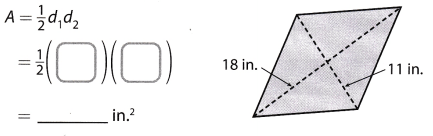Find the area of a rhombus.
d1 = 18 in, and d2 = 11 in
Use the formula for area of a rhombus.
A = $$\frac{1}{2}$$ d1d2
= $$\frac{1}{2}$$ (18) (11) Substitute.
= 99 square feet Multiply.
The area is 99 square inches.
= 99 in2

Essential Question Check-In

Question 4.
How can you find the areas of parallelograms, rhombuses, and trapezoids?
Area of a parallelogram is the product of its base and its height Mathematically it is:
Area = base × height.

Area of a trapezoid is the product of the sum of its 2 bases and its height divided by 2. Mathematically it is:
Area = $$\frac{1}{2}$$ × (b1 + b2) × h

Area of a rhombus is the product of its 2 diagonals divided by 2. Mathematically it 5: Area = $$\frac{1}{2}$$ × d1 × d2. A rhombus is a kind of a parallelogram so its area can also be evaluated by taking the product of its base and height. The choice of the formula to be used depends on the data given.

Question 5.
Rearrange the parts of the parallelogram to form a rectangle. Find the area of the parallelogram and the area of the rectangle. What is the relationship between the areas?Transforming the parallelogram to a rectangle.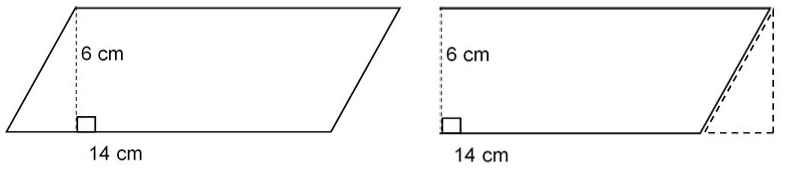Determine the area of the parallelogram and rectangle.
A = b × h formula for the area of a parallelogram
A = 14 × 6 substitute for the formula
A = 84 sq. cm. area of the parallelogram

A = 1 × W formula for the area of a rectangle
A = 14 × 6 substitute for the formula
A = 84 sq. cm. area of the rectangle
The area of the parallelogram is 84 sq. cm. while the area of the rectangle is 84 sq. cm which shows that the area of a parallelogram is equal to the area of the rectangle.

Question 6.
What is the area of a parallelogram that has a base of 12$$\frac{3}{4}$$ in. and a height of 2$$\frac{1}{2}$$ in.?
Data:
b = 12$$\frac{3}{4}$$ = 12.75
h = 2$$\frac{1}{2}$$ = 2.5
Write equation of area of a parallelogram:
Area = b × h
Substitute values:
Area = 12.75 × 2.5
Evaluate:
Area = 31.875
Area of the given parallelogram is 31.875 square inches.

Question 7.
Draw a copy of the trapezoid to form a parallelogram. Find the area of the trapezoid and the area of the parallelogram. What is the relationship between the areas?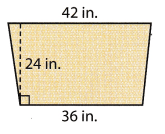Determine the area of the parallelogram and rectangle.
A = $$\frac{1}{2}$$ × h (b1 + b2) formula for the area of a trapezoid
A = $$\frac{1}{2}$$ × 24(42 + 36) substitute for the formula
A = 12 × 78 simplify
A = 936 sq. in. area of the trapezoid

b = 36 + 42 total base of the parallelogram
A = b × h formula for the area of a parallelogram
A = 78 × 24 substitute for the formula
A = 1,872 sq in. area of the parallelogram
The area of the trapezoid is 936 sq in. while the area of the parallelogram is 1,872 sq. in which shows that the area of the trapezoid is half of the area of a parallelogram.

Question 8.
The bases of a trapezoid are 11 meters and 14 meters. Its height ¡s 10 meters. What is the area of the trapezoid?
Data:
b1 = 11
b2 = 14
h = 10
Write equation of area of a trapezoid:
Area = $$\frac{1}{2}$$ × (b1 + b2) × h
Substitute values:
Area = $$\frac{1}{2}$$ × (11 + 14) × 10
Evaluate:
Area = 125
Area of the given trapezoid is 125 square meters.

Question 9.
The seat of a bench is in the shape of a trapezoid with bases of 6 feet and 5 feet and a height of 1.5 feet. What is the area of the seat?
b1 = 6
b2 = 5
h = 1.5
Write equation of area of a trapezoid:
Area = $$\frac{1}{2}$$ × (b1 + b2) × h
Substitute values:
Area = $$\frac{1}{2}$$ × (6 + 5) × 1.5
Evaluate:
Area = 8.25
Area of the seat is 8.25 square feet.

Question 10.
A kite in the shape of a rhombus has diagonals that are 25 inches long and 15 inches long. What is the area of the kite?
Find the area of a rhombus.
d1 = 25 in, and d2 = 15 in
Use the formula for area of a rhombus.
A = $$\frac{1}{2}$$d1d2
= $$\frac{1}{2}$$ (25) (15) Substitute.
= 187.5 square inches. Multiply.
Area of the kite is 187.5 square inches.
= 187.5 in2

Question 11.
A window in the shape of a parallelogram has a base of 36 inches and a height of 45 inches. What is the area of the window?
Find the area of the parallelogram.
base = 36 in, and height = 45 in
Use the formula for the area of a parallelogram. Substitute for base and height.
A = bh
= 36 × 45
= 1620
The area is 1620 square inches.
1620 in2

Question 12.
Communicate Mathematical Ideas Find the area of the figure. Explain how you found your answer.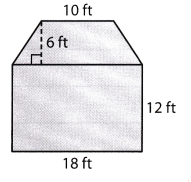SoLution to this example is given below
b1 = 10; b2 = 18; h = 6
Use the formuLa for area of a trapezoid
A = $$\frac{1}{2}$$h(b1 + b2)
= $$\frac{1}{2}$$ ∙ 6(10 + 18) Substitute
= $$\frac{1}{2}$$ ∙ 6(28) Add inside the parentheses
= 3 ∙ 28 Multiply $$\frac{1}{2}$$ and 6.
= 84 square feet Multiply
Find the area of the rectangle.
base 13 ft, and height = 9 ft
A = bh Use the formula for the area of a rectangle.
= 18 × 12 Substitute for base and height
= 216
The area is 216 square feet.
The total area is 84 ft2 + 216 ft2
= 300 ft2

Question 13.
Multistep A parking space shaped like a parallelogram has a base of 17 feet and a height is 9 feet. A car parked in the space is 16 feet long and 6 feet wide. How much of the parking space is not covered by the car?
Data of the paralleLogram shaped parking space:
b = 17
h = 9
Data of the rectangle shaped car:
l = 16
w = 6
The area not covered by car is the difference of the area of the parallelogram shaped total area and that of the rectangular shaped car so write respective formulas:
Area = (b × h) – (l × w)
Substitute values:
Area = (17 × 9) – (16 × 6)
Simplify:
Area = 153 – 96
Evaluate:
Area = 57
57 square feet of the parking space is not covered by the car.

H.O.T. Focus on Higher Order Thinking

Question 14.
Critique Reasoning Simon says that to find the area of a trapezoid, you can multiply the height by the top base and the height by the bottom base. Then add the two products together and divide the sum by 2. Is Simon correct? Explain your answer.
Write equation of area of a trapezoid:
Area = $$\frac{1}{2}$$ × (b1 + b2) × h
Rewrite:
Area = $$\frac{1}{2}$$ × (hb1 + hb2)
Or:
Area = $$\frac{\left(h b_{1}+h b_{2}\right)}{2}$$
The product hb1 is the product of the height by the top base and hb2 is the product of height by the bottom base. The plus sign shows their sum and this sum is divided by 2 to evaluate the final answer Therefore, it can be said that Simon is correct.

Question 15.
Multistep The height of a trapezoid is 8 in. and its area is 96 in2. One base of the trapezoid is 6 inches longer than the other base. What are the lengths of the bases? Explain how you found your answer.
Data:
b1 = x
b2 = x + 6
h = 8
Area = 96
Write equation of area of a trapezoid: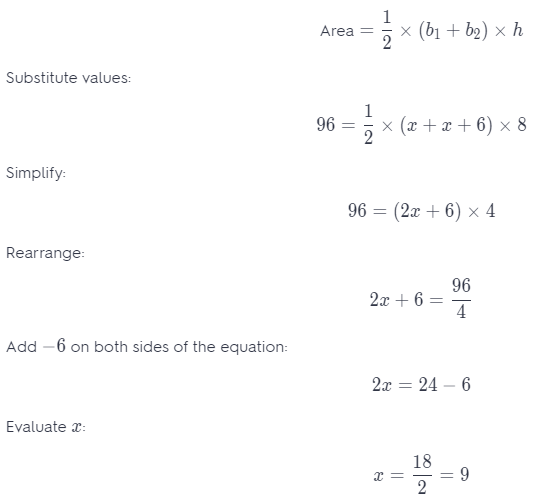The length of one of the base is 9 inches while the other is 9 + 6 = 15 inches long.

Question 16.
Critique Reasoning Find the area of the trapezoid using the formula A = $$\frac{1}{2}$$h(b1 + b2). Decompose the trapezoid into a rectangle and a triangle and find the area of each. Then find the sum of the two areas. Compare this sum with the area of the trapezoid.Determine the area of the trapezoid.
A = $$\frac{1}{2}$$ × 8 (12 + 18) substitute for the area of a trapezoid
A = 4 × 30 simplify
A = 120 sq. cm. area of the trapezoid

Determine the area of the triangle
A = $$\frac{1}{2}$$ × (6 ∙ 8) substitute for the area of a triangle
A = $$\frac{1}{2}$$ × 48 simplify
A = 24 sq. cm. area of the triangle

A = l × w formula for the area of a rectangle
A = 12 × 8 substitute for the formula
A = 96 sq. cm. area of the rectangle
A = 96 + 24 add the area of the triangle and the rectangle
A = 120 sq. cm
The area of the trapezoid is similar to the combined area of the rectangle and the triangle.

Scroll to Top